# Commutative property facts for kids

Kids Encyclopedia Facts
(Redirected from Commutative)

The commutative property of addition and multiplication tells us that it does not matter which number we add first, or multiply first. We will still get the same answer if we add them backwards. The same thing goes for multiplying backwards.

The definition of commutative property of addition is, when we substitute any number for a and b,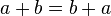$a+b=b+a$.

For example,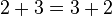$2+3=3+2$, because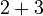$2+3$ and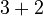$3+2$ are both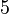$5$. It doesn't matter whether the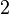$2$ or the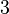$3$ comes first. 2+3=3+2 is the same as$a+b=b+a$, when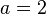$a=2$ and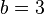$b=3$.

We can substitute fractions too.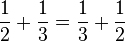$\frac{1}{2}+\frac{1}{3}=\frac{1}{3}+\frac{1}{2}$. Either way, it adds to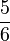$\frac{5}{6}$.

And for good mathematicians, we can substitute irrational numbers as well: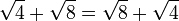$\sqrt{4}+\sqrt{8}=\sqrt{8}+\sqrt{4}$.

Some operations like dividing are not commutative. For instance, 6 divided by 3 is 2, but 3 divided by 6 is 1/2. Do not divide backwards. Dividing is not commutative. Subtracting is not commutative either: 6−2 is 4, but 2−6 is minus 4. Exponentiation is not commutative, either: For example, 2³ is 8, but 3² is 9.

In higher mathematics, there are other commutative operations besides adding and multiplying.

## Images for kidsCommutative property Facts for Kids. Kiddle Encyclopedia.